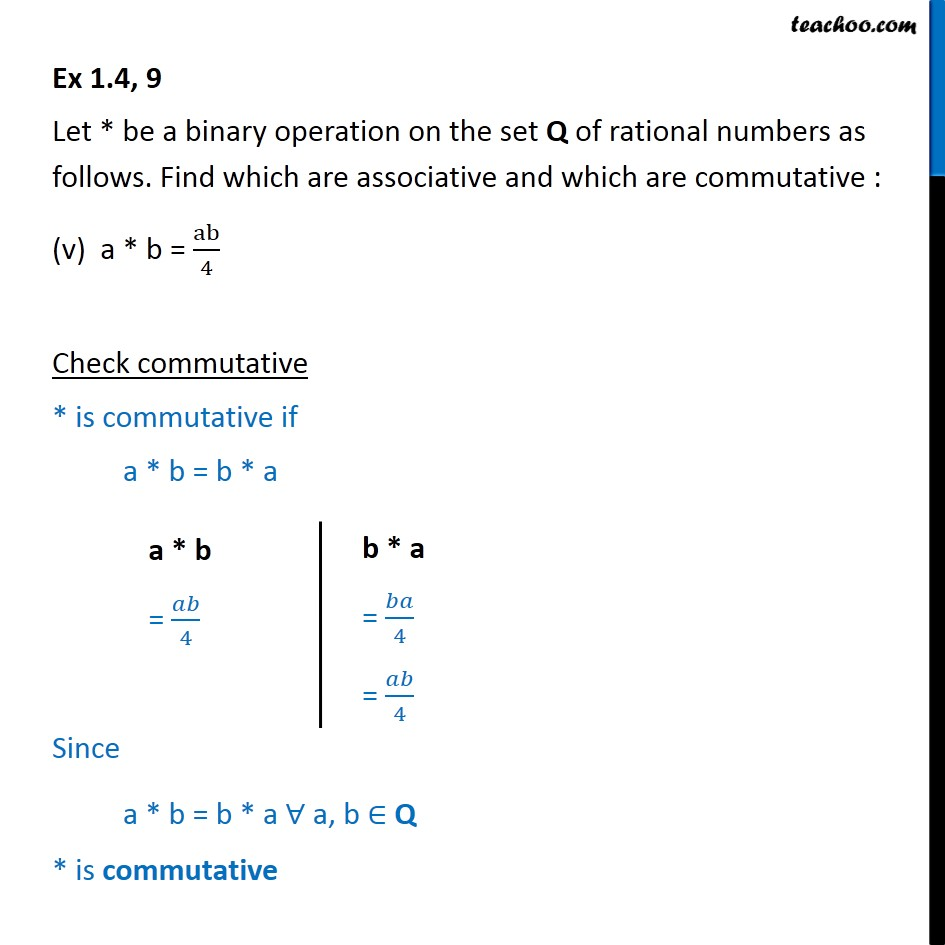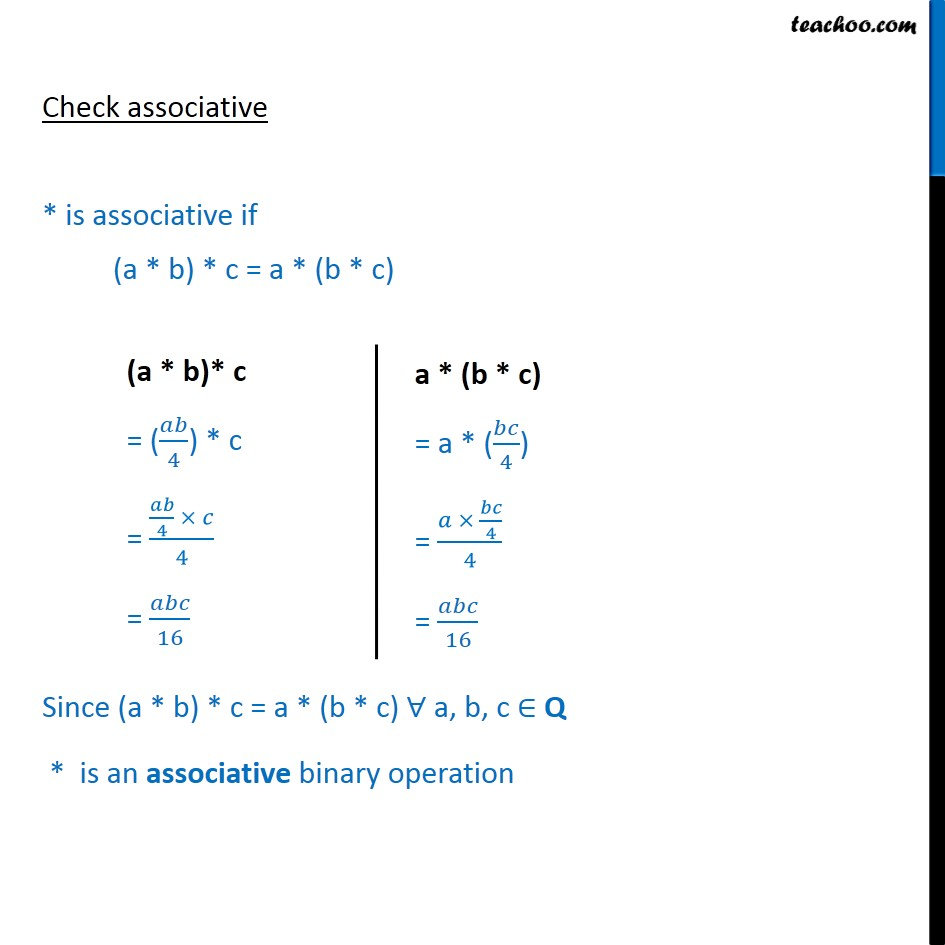Binary Operations

Chapter 1 Class 12 Relation and Functions
Serial order wiseLearn in your speed, with individual attention - Teachoo Maths 1-on-1 Class

### Transcript

Ex 1.4, 9 Let * be a binary operation on the set Q of rational numbers as follows. Find which are associative and which are commutative : (v) a * b = ab﷮4﷯ Check commutative * is commutative if a * b = b * a Since a * b = b * a ∀ a, b ∈ Q * is commutative Check associative * is associative if (a * b) * c = a * (b * c) Since (a * b) * c = a * (b * c) ∀ a, b, c ∈ Q * is an associative binary operation